The International Information Center for Structural Engineers

Wednesday, 25 January 2017 15:48cat

## Calculation Example - Calculate the member diagrams. Featured

Written by  TheStructuralEngineer.info

Calculate the member diagrams for the point load P for the pinned beam at two ends.

Solution

From the equilibrium of forces we calculate the reactions F1,F2,F3.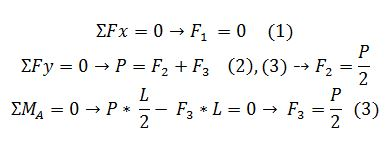We make a section 0<x<L/2 and calculate the internal forces.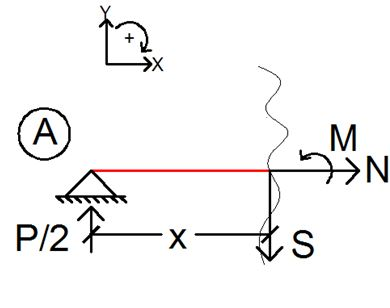We make a section L/2 <x<L and calculate the internal forces.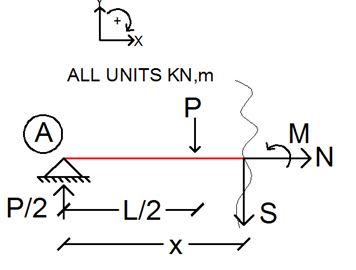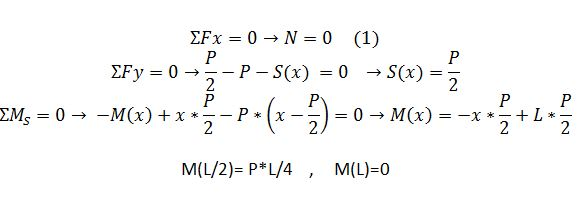Diagrams for Axial Force, Shear Force and Moment are following.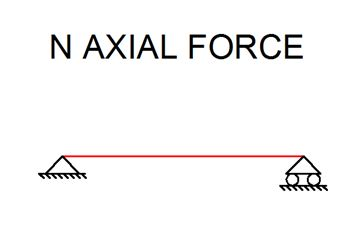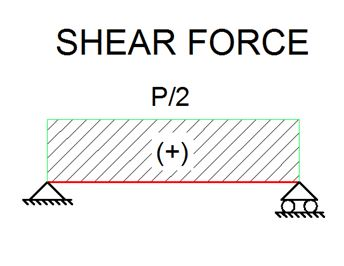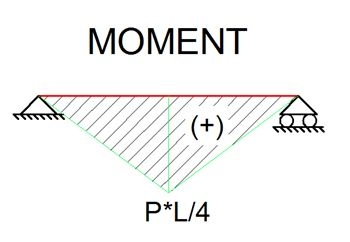• Default
• Title
• Date
• Random
• ## Keller lays foundation for Aston Martin tower

Keller business units HJ Foundation and Hayward Baker worked on the foundation system of one of Miami's most prestigious, eye-catching